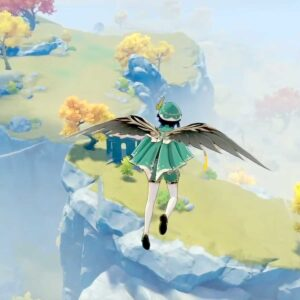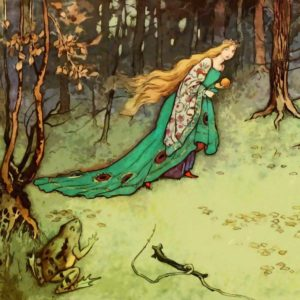# Minuet in G Major (Alternative)

23 September 2020
5
• ##### TARGET LENGTH
01:46
0
(0)

[wryd] o p [ea] s
[rd] o o
[tf] s a s d f G
[rh] o o
[es] a s d s a p
[wa] s a p o
[yI] [ro] p [wa] o
[ya] [9p] t r e
[rd] o p [ea] s
[wd] [ro] [wo]
[tf] s a s d f G
[rh] [to] r [eo] w
[es] a s d s [Qa] p
[wa] s a [rp] o
[tp] [ya] p [9o] I
[wo] 5
[wryd] o p [ea] s
[rd] o o
[tf] s a s d f G
[rh] o o
[es] a s d s a p
[wa] s a p o
[yI] [ro] p [wa] o
[ya] [9p] t r e
[rd] o p [ea] s
[wd] [ro] [wo]
[tf] s a s d f G
[rh] [to] r [eo] w
[es] a s d s [Qa] p
[wa] s a [rp] o
[tp] [ya] p [9o] I
[wo] 5
[wk] h j k h
[Qj] d f G d
[0h] [wf] G [0h] d
[eS] a S [6p]
[ep] a S d f G
[rh] [yG] [Tf]
[yG] [Qp] [eS]
[yd] 9 t
[rd] [yo] I [ro]
[tf] [uo] I [to]
[rd] [es] [wa]
[yp] o I o p
[9y] u I o [Qp] a
[0s] [ws] a s a [Qp]
[wa] d [7o] [9I]
[wryo] 9 5
[wk] h j k h
[Qj] d f G d
[0h] [wf] G [0h] d
[eS] a S [6p]
[ep] a S d f G
[rh] [yG] [Tf]
[yG] [Qp] [eS]
[yd] 9 t
[rd] [yo] I [ro]
[tf] [uo] I [to]
[rd] [es] [wa]
[yp] o I o p
[9y] u I o [Qp] a
[0s] [ws] a s a [Qp]
[wa] d [7o] [9I]
[wryo] 9 5

Rate This Music Sheet:

Average rating 0 / 5. Vote count: 0

No votes so far! Be the first to rate this music sheet.

Thank you for rating this song!

If you have any specific feedback about how to improve this music sheet, please submit this in the box below.

Minuet in G Major (Alternative) is a song by Christian Petzold. Use your computer keyboard to play Minuet in G Major (Alternative) music sheet on Virtual Piano. This is an Intermediate song and requires a lot of practice to play well. The recommended time to play this music sheet is 01:46, as verified by Virtual Piano legend, Mark Chaimbers. The song Minuet in G Major (Alternative) is classified in the genres: Classical, Germany on Virtual Piano. You can also find other similar songs using Classic Piano Songs, Happy.

• time
user
Rating
ac.
rm.
score

## Other Songs By Christian Petzold

•[utoh]| s d [yf] g [uh]| s| s| [ji]| gfgh j k [ul]| s| s| [yg]fg h g f d [tf]| g f d s [oa]| [us] d [tf] s [of]| w i [ud] y [uh]| s d [yf] g [th]| [us]| [ts]| [ji]| gfgh j k [ul]| [si] u [ys] t [yg]fg h g [rf] d [tf]| g f [ud] s [id]| [of] d [ws] a [ts]| |8| [xt]| l z x l [zr]| h j k h [le]| [tj] k [le] h [yG]| f G [d9]| [yd] f G h j k [ul]| [ok]| [jI]| [ok]| [rd]| [yG]| [oh]| w| i| [uh]| [so] a [us]| [ji]| [sp] a [si]| [uh]| [yg]| [tf]| [od] s a s d| [wo] p a s [rd] f [ge]| [tf]gf [rd]| [tf] h [s0]| [wa]| [utso]| w| 8
Level: 6
Length: 00:57
Intermediate

#### Christian Petzold

•y werty w w u tyuIo w w t ytrer trewQ werwr e |
y werty w w u tyuIo w w t ytrer trewe rewQw

Level: 1
Length: 00:40
Super Easy

## Related

•8 w u| |8 e I| |8 w u| |8 e y|h|[l8] w u|z x [C8] e I vCx z [x8] w u|z l [z8] e [yj]| |[l8] w u|l z [k8] Qlk[yj]||h [j8] q [xu]| |8 q y|h|[s8]fhlw [ut]|z x [j8]lCe [tI] vCx z [f8]hlxw [ut] z z l [d8]Gjze [yjQ]| |[sq]fjlt [pi]|l xz [wkhf] y [oja]||h [o9]dfje [yI]|||x C [h8]lzvw [ut]|v b [j8]lCe [xtI]|z|8 w [xutkf]|[znkC]|[lC8] evC[xtI]|z|[qg]jlxt [pi]|z l [wkhd] y [oa] lkj h [jfd9] e [yI]| |9 e y|x C [zvlh8] w [ut]|v b [ljC8] e [xtI]|z|8 w [xutokf]|[znC]|[tsljIC] vCx|z|[qg]jlxt [pi] z z l [wkhfd] y [oa] lkj h [pjfed] u d| |S| |[oh]|[tsol]| |[zd] [xf] [tpGC]| |[xf] [zd] [tG][ol]ax| |[zd] [sl] [ztpg]|j| |[tod]hl| |[sl] [zd] [tkdIG]| |[pj] [oh] [tjid]|[xg]|||o|[y8][wu]tos||d f [I8]eptsdG||hGf d [u8][wo]ysf||d s [y81]Ipd [ype]| |[spi84]| |s fd [oa95] sap||o [upe6] u d| |[upeT6]

Level: 7
Length: 01:57
Intermediate

#### Yu-Peng Chen

•[x6]fj[x0]fj[xe]fj [x6]fj[z0]fj[le]fj [l6]sf[k0]sf[je]sf [j6]sf[l0]fj[xe]jl [b6]jz[b9]jz[eb]jz [b6]jz[v9]jz[ec]jz [c6]fj[x9]fj[ze]fj [z6]fj[x9]fj[ec]fj [x0]Hk[rc]Hk[xu]Hk [x0]Hk[rV]Hk[xu]Hk [x6]fj[z0]fj[le]fj [l6]sf[k0]sf[je]sf [k0]df[rk]df[uk]df [k0]df[rl]df[uk]df [je]sf[j0]sf[j8]sf[sjf6]
Level: 7
Length: 00:35
Intermediate
##### Romance d’Amour
•[r6] t r t r t e r e r t e [t64] y t y t y r t r t y u [r6] t r t r t e r e r t e [t64] y t y t y r t r t y u [ra6] t [r0] t [re] t [ue] r e r t e [t6] y [tq] y [te] y r [ti] [ro] [tp] [ya] [us] [ra6] t [r0] t [re] t [ue] r e r t e [t6] y [tq] y [te] y r [ti] [ro] [tp] [ya] [us] [f2] u [qie9] u [yqie] p [ud2] i [yqe9] u [yqpe]|[f5] u [wri9] u [ywri] p [ud5] i [ywrh] u [ywr] s [a1] r [t80] r [wt0] u [r1] t [e80] r [w0]|[a3] u [spe0] u [yeda] p [uf3] O [raW0] u [rWO0]|[i4]pfy [uq8] p [yqe] u [i4]pdy [uq8] p [tqe]|[oda5]|[wroa9]|[ywsr]|[od5]|[wsr9]|[ywr] a [oa1] y [wut] a [r0] y [uo1] y [wut] a [r0] y [ut6]|[pe0]|[tea]|[s6]|[wa0]|[tpe]|[f4]jx|[tqe]|[ute]|[zjd4]|[tqe]|[ute]|[o5]ad|[wrkda9]|[ywsl]|[zhd5]|[wsrl9]|[yw] [ka] [kfa1]|[wr0]|[wut]|[ohfa1]|[wr0]|[wut]|[ka3]|0 r [pje] r [O0][ra][uf]H| |[r60] t r t r t [e0] r e r t e [tq64] y t y t y [rq] t r t r t [r60] t r t r t [e0] r e r t e [tq64] y t y t y [rq] t r t r|[ka6] u [ta0] s [uea] u [uf6] u [ta0] s [upe] u 6 i [tqa] s [iea] i 6 [ig] [tqoh] [pj] [kiea] [sl] [ka6] u [ta0] s [uea] u [uf6] u [ta0] s [upe] u 6 i [tqa] s [iea] i 6 [ig] [tqoh] [pj] [kiea] [sl] [xjf2] u [qi9] p [uqe] i [zd2] u [qi9] p [qpje]|[xjf5] u [wri9] p [ywur] i [zd5] 9 [wvh] e [wr] [sl] [ka1] r [t80] u [wr0] t [e1] r [w80] e [wr0]|[ka3] 7 [sl0] e [zrd] e [f3]Hkx [urW]|0|[tqed9] f d f d f [rqd9] f d f [ed] f [tqed9] f d f d f [rqd9] f h z [wk7]|[wrd80] f d f d f [ed80] f d f [d0] f [wd80] f d f d f d f k f d|[si9] q e y i|[ia9] q e y [pi]|[si5] 9 w e r|[ia5] 9 w e [yro]|[uta1] 5 8 w u|[tp1] 5 8 w [ut]|[ro51] 8 w t u o [ua]

Level: 7
Length: 02:49
Intermediate